# 分布式系统中的时钟与一致性解读（上）

ywz888

0收藏

●   1 物理时钟与问题
●   2 逻辑时钟 Logicl Clock，LC
●   3 向量时钟 Vector Clock，VC
●   4 TrueTime, TT
●   5 混合逻辑时钟 Hybrid Logical Clocks，HLC
■   5.1 概念预介绍
■   5.2 算法介绍
●   6 一致性
■   6.1 理论中的一致性
■   6.2 分布式系统中实现的一致性
●   7 多说一点

1 物理时钟与问题

2 逻辑时钟 Logical Clock，LC

●   捕获因果关系（Happened before relation，符号是：->）：a -> b，代表a先于b发生，a, b代表事件

●   逻辑时钟（Logical Clock），用 C 来表示逻辑时钟，逻辑时钟的标准是：

■   [C1]: Ci(a) < Ci(b), [ 说明：Ci -> 代表逻辑时钟, 如果 a 发生在 b 之前, 那么事件a的逻辑时间要小于b的逻辑时间， i 代表在 i 这个特定的进程中 ]

■   [C2]: Ci(a) < Cj(b), [ 说明：代表不同进程发生的事件逻辑时钟的值 Ci(a) 小于 Cj(b)  ]

●   进程（Process）：Pi

●   事件（Event）：Eij，这里 i 是进程号， Eij 是进程号为 i 的第 j 个事件

●   tm：消息m的向量时间跨度，表示在进程之间传递

●   Ci 向量时钟关联到进程 Pi , 他的第 j 个元素是 Ci[j] ，包含进程  Pi 当前时间的最新值在进程 Pj 中

●   d: 漂移时间，通常是1（即每有新事件发生，进程的逻辑时钟递增1）

●   [IR1]: 如果 a -> b [ 说明：在同一个进程中，事件a先于事件b发生 ] ， 那么， Ci(b) = Ci(a) + d
●   [IR2]: Cj = max(Cj, tm + d) [ 说明：如果存在多进程，在进程之间发送事件，tm 等于 Ci(a) 的值，Cj 等于Cj和 tm + d 中的最大值 ] ]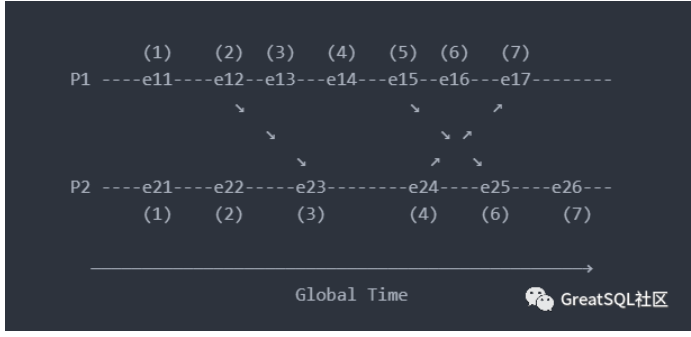●   初始值为1，因为它是第一个事件，并且在起点没有传入值（由其他线程传入的逻辑时钟）
■   e11 = 1
■   e21 = 1
●   下一个点的值将继续增加 d （d = 1），如果没有传入的值，即适用[IR1]
■   e12 = e11 + d = 1 + 1 = 2
■   e13 = e12 + d = 2 + 1 = 3
■   e14 = e13 + d = 3 + 1 = 4
■   e15 = e14 + d = 4 + 1 = 5
■   e16 = e15 + d = 5 + 1 = 6
■   e22 = e21 + d = 1 + 1 = 2
■   e24 = e23 + d = 3 + 1 = 4
■   e26 = e25 + d = 6 + 1 = 7

■   e17 = max(7, 5) = 7, [ e16 + d = 6 + 1 = 7, e24 + d = 4 + 1 = 5, 7与5选择最大值5 ]
■   e23 = max(3, 3) = 3, [ e22 + d = 2 + 1 = 3, e12 + d = 2 + 1 = 3, 3与3选择最大值3 ]
■   e25 = max(5, 6) = 6, [ e24 + 1 = 4 + 1 = 5, e15 + d = 5 + 1 = 6, 5与6选择最大值6 ]

■   适用规则[IR1]时, 如果 a -> b, 那么 C(a) < C(b) 正确，即在同一个线程中，事件 a 先于事件 b 发生，那么一定 C(a) < C(b)
■   适用规则[IR2]时, 如果 a -> b, 那么 C(a) < C(b) 可能正确也可能不正确。即在不同线程中，线程1中的事件 a 的逻辑时钟与线程2中的事件 b 的逻辑时钟大小关系不确定，如下图中的e21，e31的逻辑时钟先后无法确定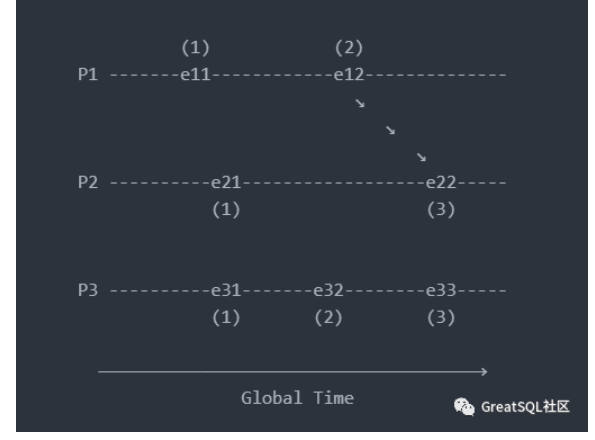3 向量时钟 Vector Clock，VC
Lamport逻辑时钟虽然解决了存在依赖关系的事件时序，但是不同节点同时发生的事件不能很好的进行排序。向量时钟对逻辑时钟进行了继承与发展，它的时钟更新逻辑与逻辑时钟一样。不同在于逻辑时钟只有存在依赖关系的事件才会在节点间发送，而向量时钟则是每一个事件发生后，都会向当前所有节点发送（广播），其他节点接收后，更新本地逻辑时钟，这样就解决了所有事件的依赖关系与时序。

●   对于进程 i 来说，Ti[i] 是进程 i 本地的逻辑时间
●   当进程 i 有新的事件发生时，Ti[i] = Ti[i] + 1
●   当进程 i 发送消息时将它的向量时间戳(MT=Ti)附带在消息中。
●   接受消息的进程 j 更新本地的向量时间戳：TJ[k] = max(Tj[k], MT[k])， for k = 1 to N。（MT即消息中附带的向量时间戳）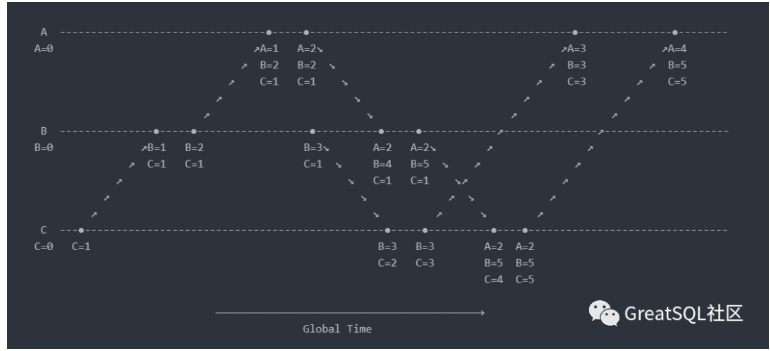4 TrueTime, TT
TrueTime 是 Google 公司提出的概念，用于在分布式系统中提高物理时钟的可靠性。确切的说这是物理时钟 + 算法，是一种偏向硬件的解决方案，可以分配统一的、具有可比性的 timestamp 范围。在 Google 的 Spanner 上得到了应用。时钟硬件由GPS时钟和原子钟组成，因为GPS的天线或者接收故障与原子钟的频率导致漂移的故障原因不交叉，可以提高时钟的可靠性。在部署上支持全球部署，在每个数据中心都会部署若干time master机器，机器之间会通信校验时间，用于物理时间同步，能够保证TrueTime的误差范围 ε 是1ms到7ms 之间。相对于 NTP 的误差范围 100 毫秒大大降低，这是借助硬件和算法实现的。

TrueTime API的直接数据来源是设备上的local clock. 所以TrueTime的误差来源有:

●   从time master同步时的网络延迟, 导致的误差大概是1ms
●   local clock的漂移, 校准后的一瞬间是0, 校准前的一瞬间是最大值, 范围在0~6ms

TrueTime提供了三个API来操作时间：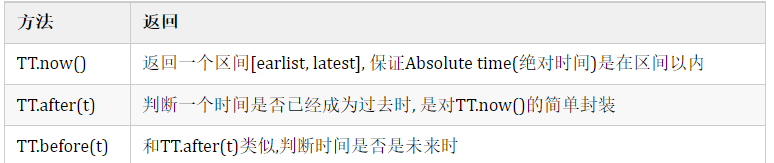●   Start：提交事务Ti时，事务协调者必须选择一个大于等于TT.now().latest的时间作为提交时间戳si

S1 的时间比绝对时间快5ms，即 T = Tabs + 5
S2 的时间比绝对时间慢4ms，即 T = Tabs - 4
S3 的时间比绝对时间慢2ms，即 T = Tabs - 2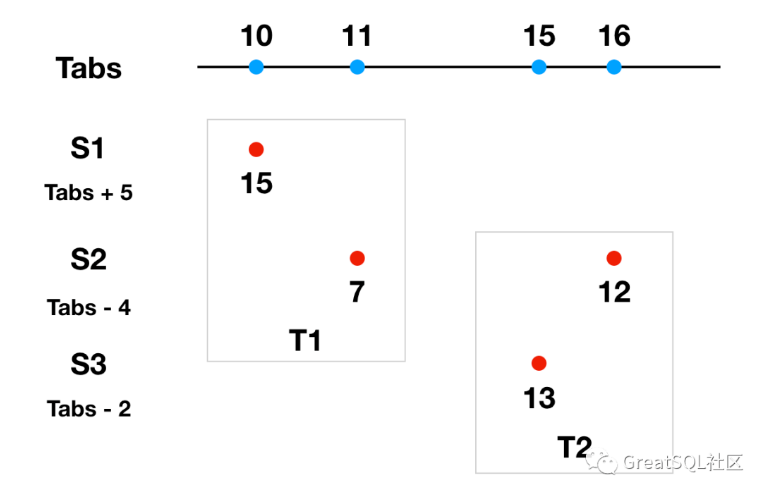S2 作为协调者发起分布式事务T1，参与者 S1、S2，S1 执行 T1 本地分支事务时间为 15ms （此时 Tabs 为 10ms）, S2 执行 T1 本地分支事务的本地时间是 7ms（此时 Tabs 为 11ms），S2作为协调者需要选择参与者中时间最大成员时间作为提交时间，所以选择S1的 15ms 作为提交时间。

S2作为协调者又开启了分布式事务 T2，参与者包括 S2、S3，S3 执行本地分支事务的时间是 13ms（此时 Tabs 为 15ms），S2 执行本地分支事务的时间是 12ms（此时 Tabs 为 16ms）。S2 还是作为协调者，提交事务时选择了 13ms 作为整个事务的执行时间。

S2 作为协调者在提交 T1 选择提交时间 s1 时，根据 Start 规则需要大于所有事务参与者（此时是S1、S2）最大的本地时间，并且需要大于 S2 本地的 TT.now().lastest （即TT.now() + ε），所以 s1 = max(15, 7 + 7) = 15ms。
s1确定后，根据 Commit Wait 规则，还要等待 TT.after(15) 为true后（即在误差时间 7ms 后，才能确认 s1 是过去时间，此时S2本地时间是 23ms）才能提交数据。也就是 TT.now() 是在[16, 30]区间的，S2 的本地时间是 23ms ，绝对时间 27ms 提交数据。

S2 作为协调者在提交 T2 选择提交时间 s2 时，根据 Start 规则得出 s2 = s2 = max(13, 12 + 7) = 19ms。
s2确定后，根据 Commit Wait 规则，还要等待 TT.after(19) 为true后（即在误差时间 7ms 后，才能确认 s2 是过去时间，此时S2本地时间是 27ms）才能提交数据。也就是 TT.now() 是在[20, 34]区间的，S2 的本地时间是 27ms ，绝对时间 31ms 提交数据。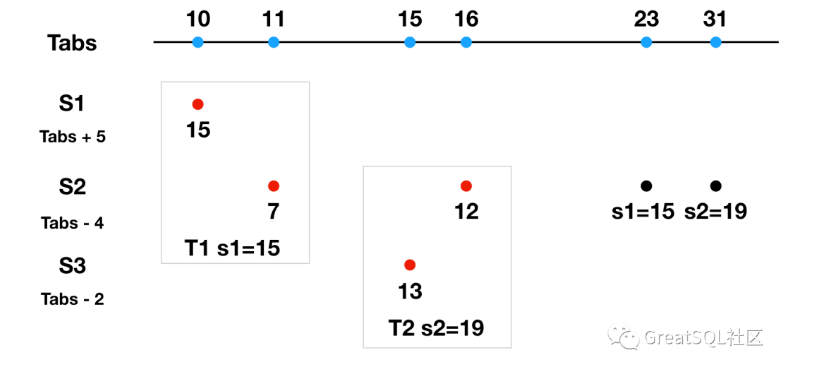http://yang.observer/2020/11/02/true-time/帖子
视频
声望
粉丝
最近发布
社区精华内容